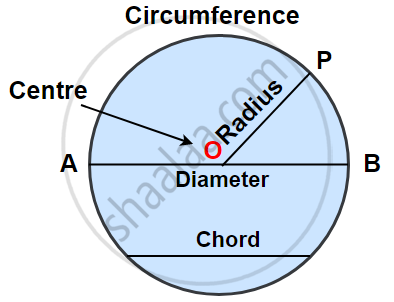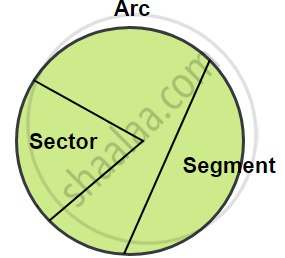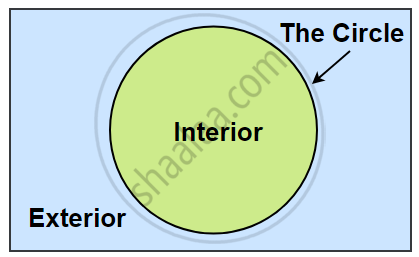# Concept of Circle - Centre, Radius, Diameter, Arc, Sector, Chord, Segment, Semicircle, Circumference, Interior and Exterior, Concentric Circles

## Definition

• Circle: The collection of all the points in a plane, which are at a fixed distance from a fixed point in the plane, is called a circle.
• Centre of a circle: The fixed point is called the centre of the circle.
• Radius of a circle: The line segment joining the centre and any point on the circle is called the radius of the circle.
• Circumference of a circle: The Perimeter of the circle is called the circumference.
• Chord of a circle: The line segment, joining any two points on the circumference of the circle, is called a chord.
• Sector of a circle: A sector is a region in the interior of a circle enclosed by an arc on one side and a pair of radii on the other two sides.
• Segment of a circle: A segment of a circle is a region in the interior of the circle enclosed by an arc and a chord.
• Semi-circle: A semi-circle is half of a circle, with the endpoints of diameter as part of the boundary.

## Notes

### Circles:

• The collection of all the points in a plane, which are at a fixed distance from a fixed point in the plane, is called a circle.• The fixed point is called the centre of the circle.

• The fixed distance is called the radius of the circle.
The line segment joining the centre and any point on the circle is also called a radius of the circle. That is, ‘radius’ is used in two senses in the sense of a line segment and also in the sense of its length.

• The Perimeter of the circle is called the circumference.

• The line segment, joining any two points on the circumference of the circle, is called a chord.

• A chord, which passes through the centre of the circle is called the diameter and is the largest chord of the circle which is equal to two times the radius.• A sector is a region in the interior of a circle enclosed by an arc on one side and a pair of radii on the other two sides.
• A segment of a circle is a region in the interior of the circle enclosed by an arc and a chord.

• A diameter of a circle divides it into two equal parts; each part is a semi-circle. A semi-circle is half of a circle, with the endpoints of diameter as part of the boundary.

• A circle divides the plane on which it lies into three parts.
They are:
(i) inside the circle, which is also called the interior of the circle;
(ii) the circle and
(iii) outside the circle, which is also called the exterior of the circle, which is also called the exterior of the circle. The circle and its interior make up the circular region.If you would like to contribute notes or other learning material, please submit them using the button below.

### Shaalaa.com

Circle - Radius and Diameter [00:14:59]
S
0%